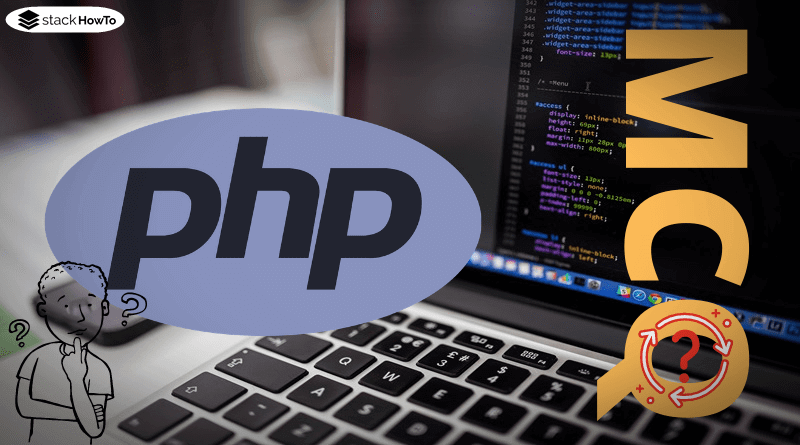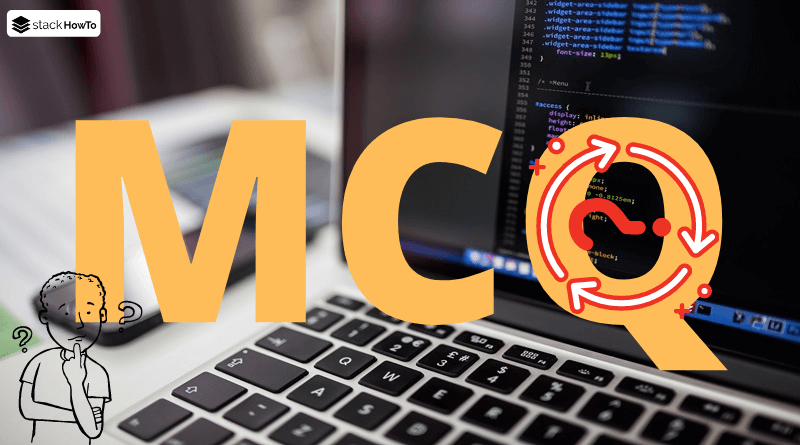php

# PHP References: When to Use Them, and How They Work

If you pass a variable of a primitive data type (Boolean, Integer, Float, String, Array) to a function or assign it to another variable, then by default in PHP only the value (i.e. the content) is copied. If one would make changes to the new variable, the value of the old variable would remain unchanged. If you want the old variable to be changed as well, you have to use references instead. These are alternative names for the same variable.

###### Example 1: A simple reference

The following example shows first how variables behave without reference (section “without reference”). Then the behavior with reference is shown. Here the variable \$x is initialized with 1 and \$y is defined as a reference to \$x. Every change to \$y now also affects \$x, which was not the case before.

```<?php
// without reference
\$x = 1;
\$y = \$x;
\$y = \$y + 5;

echo("x: \$x, y: \$y \n");

// with reference
\$x = 1;
\$y = &\$x;
\$y = \$y + 5;

echo("x: \$x, y: \$y \n");
?>```

Output:

```x: 1, y: 6
x: 6, y: 6```

###### Example: Reference over multiple variables

References can also have effects over multiple variables:

```<?php
\$x = 1;
\$y = &\$x;
\$z = &\$y;
\$u = &\$z;
\$w = &\$u;
\$w = 'example';

echo("x: \$x \n");
?>```

Output:

`x: example`PHP Questions and Answers – Object-Oriented OOP – Part 1This collection of PHP Multiple Choice Questions and Answers (MCQs): Quizzes & Practice Tests with Answer focuses on “Object-Oriented OOP”.   1. The concept of…Read More
###### Example: Passing a reference to a function

In this example a function is defined, which takes a reference as variable and increases this variable by one. Although the result is not returned by “return”, the variable still contains the value increased by one.

```<?php
function increment(&\$var) {
\$var = \$var + 1;
}

\$a = 1;
increment(\$a);
echo(\$a);
?>```

Output:

`2`

Usually, it is already specified in the definition of the function whether the parameters should be references or not. But it is also possible to ignore this and pass a reference, although this is not intended. However, this procedure is outdated and therefore generates a deprecated warning when used.

```<?php
function increment(\$var) {
\$var = \$var + 1;
}

\$a = 1;
increment(&\$a);
echo(\$a);
?>```

Output:

```Deprecated:  Call-time pass-by-reference has been deprecated; If you would like to pass it by reference, modify the declaration of increment().  If you would like to enable call-time pass-by-reference, you can set allow_call_time_pass_reference to true in your INI file in ...\main.php on line 7
2```

###### Example: Objects are transferred as a reference by default

While primitive data types are copied by default, variables with objects are always references. You can therefore pass them to functions or other variables and change them – this has effects on all variables, because internally they point to the same object and the changes are made to this object.

```<?php
class Test {
public \$a = 0;
}

function increment(Test \$obj) {
\$obj->a = \$obj->a + 1;
}

\$test = new Test();
echo(\$test->a . "\n");

// Pass \$test to another function and increase the value in it
increment(\$test);
increment(\$test);
increment(\$test);
echo(\$test->a . "\n");

// Pass \$test to another variable and use it to increase the value
\$b = \$test;
\$b->a = \$b->a + 5;
echo(\$test->a . "\n");
echo(\$b->a);
?>```

Output:

```0
3
8
8```MCQPractice competitive and technical Multiple Choice Questions and Answers (MCQs) with simple and logical explanations to prepare for tests and interviews.Read More